# 损失规避行为下的一般保险公司最优投资再保险问题Optimal Investment and Reinsurance Problem for a General Insurance Company under Behavior of Loss Aversion

DOI: 10.12677/SA.2020.92017, PDF, HTML, XML, 下载: 156  浏览: 231

Abstract: In the paper, we study the optimal investment reinsurance problem for general insurance companies (insurance company and reinsurance company) based on behavior of loss aversion. Managers manage risk by purchasing proportional reinsurance and investing in financial markets. Assume that the claim process is described by a Brownian motion with drift. The premium rate is calculated through the expectation principle. Both insurance company and reinsurance company are allowed to invest in risk-free assets and risky assets. The price process of risk assets is subject to a jump-diffusion process. Our goal is to maximize the common interests of insurance company and reinsurance company, i.e. Maximizing the weight sum of the wealth process. Using the martingale method, we derive the closed expression of the optimal wealth process weight and its corresponding optimal investment reinsurance strategies. Finally, numerical examples are provided to show the impact of model parameters on the optimal strategies.

1. 引言

2. 模型建立

$\left(\Omega ,F,P\right)$ 是完备的概率空间， ${\left\{{F}_{t}\right\}}_{0\le t\le T}$ 是由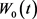${W}_{1}\left(t\right)$${W}_{2}\left(t\right)$${B}_{1}\left(t\right)$${B}_{2}\left(t\right)$ 五个独立的、标准的一维布朗运动所生成的过滤，表示当前时刻t获得的所有信息, 且假设所有在t时刻作的决定都是基于这些信息。此外，在本文中假设所有的随机变量和随机过程都可以被很好的定义且都是关于 ${\left\{{F}_{t}\right\}}_{0\le t\le T}$ 是适应的。

2.1. 盈余过程

$\text{d}R\left(t\right)=c\text{d}t+\underset{i=1}{\overset{K\left(t\right)}{\sum }}{Y}_{i}$

$\text{d}H\left(t\right)=A\text{d}t+B\text{d}{W}_{0}\left(t\right)+\gamma \text{d}N\left(t\right),$

$A+{\lambda }_{N}\gamma ={\lambda }_{K}{\mu }_{Y}$, ${B}^{2}+{\lambda }_{N}{\gamma }^{2}={\lambda }_{K}{\sigma }_{Y}^{2}$.

$\begin{array}{c}\text{d}{R}_{1}\left(t\right)=\left\{\left[\left(1-{\pi }_{q}\left(t\right)\right)+\left(\eta -\theta {\pi }_{q}\left(t\right)\right)\right]{\lambda }_{K}{\mu }_{Y}-\left(1-{\pi }_{q}\left(t\right)\right)A-{\lambda }_{N}\gamma \left(1-{\pi }_{q}\left(t\right)\right)\right\}\text{d}t\\ \text{\hspace{0.17em}}\text{\hspace{0.17em}}-\left(1-{\pi }_{q}\left(t\right)\right)B\text{d}{W}_{0}\left(t\right)-\left(1-{\pi }_{q}\left(t\right)\right)B\text{d}\stackrel{˜}{N}\left(t\right),\end{array}$

$\text{d}{R}_{2}\left(t\right)=\left\{\left(1+\theta \right){\lambda }_{K}{\mu }_{Y}{\pi }_{q}\left(t\right)-{\pi }_{q}\left(t\right)A-{\lambda }_{N}\gamma {\pi }_{q}\left(t\right)\right\}\text{d}t-{\pi }_{q}\left(t\right)B\text{d}{W}_{0}\left(t\right)-{\pi }_{q}\left(t\right)\gamma \text{d}\stackrel{˜}{N}\left(t\right).$

2.2. 金融市场

$\frac{\text{d}{S}_{0}\left(t\right)}{{S}_{0}\left(t\right)}=r\text{d}t,\text{\hspace{0.17em}}\text{\hspace{0.17em}}{S}_{0}\left(0\right)=0,$

$\frac{\text{d}{S}_{1}\left(t\right)}{{S}_{1}\left(t\right)}={a}_{1}\left(t\right)\text{d}t+{b}_{1}\left(t\right)\text{d}{W}_{1}\left(t\right)+\underset{i=1}{\overset{{K}_{1}\left(t\right)}{\sum }}{Y}_{i}^{1},$

$\frac{\text{d}{S}_{\text{2}}\left(t\right)}{{S}_{\text{2}}\left(t\right)}={a}_{\text{2}}\left(t\right)\text{d}t+{b}_{\text{2}}\left(t\right)\text{d}{W}_{\text{2}}\left(t\right)+\underset{i=1}{\overset{{K}_{\text{2}}\left(t\right)}{\sum }}{Y}_{i}^{\text{2}},$

$\text{d}\underset{i=1}{\overset{{K}_{j}\left(t\right)}{\sum }}{Y}_{i}^{j}={\lambda }_{j}{\mu }_{j}\text{d}t-\sqrt{{\lambda }_{j}}{\sigma }_{j}\text{d}{B}_{j}\left(t\right),\text{\hspace{0.17em}}\text{\hspace{0.17em}}j=1,2.$

$\frac{\text{d}{S}_{1}\left(t\right)}{{S}_{1}\left(t\right)}=\left[{a}_{1}\left(t\right)+{\lambda }_{1}{\mu }_{1}\right]\text{d}t+{b}_{1}\left(t\right)\text{d}{W}_{1}\left(t\right)-\sqrt{{\lambda }_{1}}{\sigma }_{1}\text{d}{B}_{1}\left(t\right),$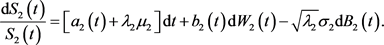${X}_{1}^{\pi }\left(t\right)$${X}_{\text{2}}^{\pi }\left(t\right)$ 分别是保险公司和再保险公司的总财富过程。设 ${\pi }_{\text{1}}\left(t\right)$ 是保险公司投资在风险资产 ${S}_{1}\left(t\right)$ 的总金额， ${\pi }_{\text{2}}\left(t\right)$ 是再保险公司投资在风险资产 ${S}_{\text{2}}\left(t\right)$ 的总金额，因此 ${X}_{\text{1}}^{\pi }-{\pi }_{1}\left(t\right)$${X}_{\text{2}}^{\pi }-{\pi }_{\text{2}}\left(t\right)$ 分别是保险公司和再保险公司分别投资在无风险资产 ${S}_{\text{0}}\left(t\right)$ 的金额。假设一个贸易策略可以由一个三元组 $\pi \left(t\right):=\left({\pi }_{q}\left(t\right),{\pi }_{1}\left(t\right),{\pi }_{2}\left(t\right)\right)$ 表示。在策略 $\pi \left(t\right)$ 下，保险公司的财富过程 ${X}_{1}^{\pi }\left(t\right)$ 和再保险公司的财富过程 ${X}_{\text{2}}^{\pi }\left(t\right)$ 的价格动态过程分别服从

$\begin{array}{l}\text{d}{X}_{1}^{\pi }\left(t\right)=\left\{r{X}_{1}^{\pi }\left(t\right)+{\pi }_{1}\left(t\right)\left({a}_{1}\left(t\right)+{\lambda }_{1}{\mu }_{1}-r\right)+\left[\left(1-{\pi }_{q}\left(t\right)\right)+\left(\eta -\theta {\pi }_{q}\left(t\right)\right)\right]{\lambda }_{K}{\mu }_{Y}\\ \text{ }\text{ }\text{ }\text{ }\text{ }\text{ }\text{ }\text{ }\text{ }\text{ }\text{ }\text{ }\text{ }\text{ }\text{ }\text{ }\text{ }\text{ }\text{ }\text{ }\text{ }\text{ }\text{ }\text{ }\text{ }\text{\hspace{0.17em}}\text{ }\text{ }\text{ }\text{ }\text{ }\text{ }\text{\hspace{0.17em}}\text{ }\text{ }\text{ }\text{ }\text{ }-\left(1-{\pi }_{q}\left(t\right)\right)A-{\lambda }_{N}\gamma \left(1-{\pi }_{q}\left(t\right)\right)\right\}\text{d}t-\left(1-{\pi }_{q}\left(t\right)\right)B\text{d}{W}_{0}\left(t\right)\\ \text{ }\text{ }\text{ }\text{ }\text{ }\text{ }\text{ }\text{ }\text{ }\text{ }\text{ }\text{ }\text{ }\text{ }\text{ }\text{ }\text{ }\text{ }\text{ }\text{ }\text{ }\text{ }\text{ }\text{ }\text{ }\text{\hspace{0.17em}}\text{\hspace{0.17em}}\text{ }\text{ }\text{ }\text{ }\text{ }\text{ }\text{ }\text{ }\text{ }\text{ }\text{ }+{\pi }_{1}\left(t\right){b}_{1}\left(t\right)\text{d}{W}_{0}\left(t\right)-{\pi }_{1}\left(t\right)\sqrt{{\lambda }_{1}}{\sigma }_{1}\text{d}{B}_{1}\left(t\right)-\left(1-{\pi }_{q}\left(t\right)\right)\gamma \text{d}\stackrel{˜}{N}\left(t\right)\end{array}$ (1)

$\begin{array}{c}\text{d}{X}_{2}^{\pi }\left(t\right)=\left\{r{X}_{2}^{\pi }\left(t\right)+{\pi }_{2}\left(t\right)\left({a}_{2}\left(t\right)+{\lambda }_{2}{\mu }_{2}-r\right)+\left(1+\theta \right){\lambda }_{K}{\mu }_{Y}{\pi }_{q}\left(t\right)-{\pi }_{q}\left(t\right)A-{\lambda }_{N}\gamma {\pi }_{q}\left(t\right)\right\}\text{d}t\\ \text{\hspace{0.17em}}\text{\hspace{0.17em}}-{\pi }_{q}\left(t\right)B\text{d}{W}_{0}\left(t\right)+{\pi }_{2}\left(t\right){b}_{2}\left(t\right)\text{d}{W}_{2}\left(t\right)-{\pi }_{2}\left(t\right)\sqrt{{\lambda }_{2}}{\sigma }_{2}\text{d}{B}_{2}\left(t\right)-{\pi }_{q}\left(t\right)\gamma \text{d}\stackrel{˜}{N}\left(t\right),\end{array}$ (2)

${Y}^{\pi }\left(t\right)={\alpha }_{1}{X}_{1}^{\pi }\left(t\right)+{\alpha }_{2}{X}_{2}^{\pi }\left(t\right)$ (3)

$\begin{array}{l}\text{d}{Y}^{\pi }\left(t\right)=\left\{r{Y}^{\pi }\left(t\right)+{\alpha }_{1}\left(1+\eta \right){\lambda }_{K}{\mu }_{Y}-{\alpha }_{1}\left(A+{\lambda }_{N}\gamma \right)-\left({\alpha }_{1}-{\alpha }_{2}\right){\lambda }_{K}{\mu }_{Y}{\pi }_{q}\left(t\right)\\ \text{ }\text{ }\text{ }\text{ }\text{ }\text{ }\text{ }\text{ }\text{ }\text{ }\text{ }\text{ }\text{ }\text{ }\text{ }\text{ }\text{ }\text{ }\text{ }\text{ }\text{ }\text{ }\text{ }\text{ }\text{ }\text{ }\text{ }\text{ }\text{ }\text{\hspace{0.17em}}\text{ }\text{ }\text{ }\text{ }\text{ }\text{ }+\underset{j=1}{\overset{2}{\sum }}{\alpha }_{j}{\pi }_{j}\left(t\right)\left({\alpha }_{j}\left(t\right)+{\lambda }_{j}{\mu }_{j}-r\right)-\left({\alpha }_{1}-{\alpha }_{2}\right)\theta {\lambda }_{K}{\mu }_{Y}{\pi }_{q}\left(t\right)+\left({\alpha }_{1}-{\alpha }_{2}\right){\pi }_{q}\left(t\right)A\\ \text{\hspace{0.17em}}\text{ }\text{ }\text{ }\text{ }\text{ }\text{ }\text{ }\text{ }\text{ }\text{ }\text{ }\text{ }\text{ }\text{ }\text{ }\text{ }\text{ }\text{ }\text{ }\text{ }\text{ }\text{ }\text{ }\text{ }\text{ }\text{ }\text{ }\text{ }\text{ }\text{ }\text{ }\text{ }\text{ }\text{ }\text{ }+\left({\alpha }_{1}-{\alpha }_{2}\right){\lambda }_{N}\gamma {\pi }_{q}\left(t\right)\right\}\text{d}t-\left[{\alpha }_{1}-\left({\alpha }_{1}-{\alpha }_{2}\right){\pi }_{q}\left(t\right)\right]B\text{d}{W}_{0}\left(t\right)+\underset{j=1}{\overset{2}{\sum }}{\alpha }_{j}{\pi }_{j}\left(t\right){b}_{j}\left(t\right)\text{d}{W}_{j}\left(t\right)\\ \text{ }\text{ }\text{ }\text{ }\text{ }\text{ }\text{ }\text{ }\text{ }\text{ }\text{ }\text{ }\text{ }\text{ }\text{ }\text{ }\text{ }\text{ }\text{ }\text{ }\text{ }\text{\hspace{0.17em}}\text{ }\text{ }\text{ }\text{ }\text{ }\text{ }\text{ }\text{ }\text{ }\text{ }\text{ }\text{ }\text{ }-\underset{j=1}{\overset{2}{\sum }}{\alpha }_{j}{\pi }_{j}\left(t\right)\sqrt{{\lambda }_{j}}{\sigma }_{j}\text{d}{B}_{j}\left(t\right)-\left[{\alpha }_{1}-\left({\alpha }_{1}-{\alpha }_{2}\right){\pi }_{q}\left(t\right)\right]\gamma \text{d}\stackrel{˜}{N}\left(t\right),\end{array}$ (4)

(1) $\pi \left(t\right):=\left({\pi }_{q}\left(t\right),{\pi }_{1}\left(t\right),{\pi }_{2}\left(t\right)\right)$ 是可测的。

(2) 对于任意 $t\in \left[0,T\right]$${\pi }_{q}\in \left[0,1\right]$${\pi }^{\text{2}}\left(t\right)={\pi }_{q}^{2}\left(t\right)+{\pi }_{1}^{2}\left(t\right)+{\pi }_{2}^{2}\left(t\right)$

(3) 方程(4)有唯一解。

3. S-型效用函数的优化问题

$U\left(x\right)=\left\{\begin{array}{l}{U}_{1}\left(x\right),\text{ }\text{ }\text{ }\text{ }\text{ }\text{ }\text{ }x>0,\\ {U}_{2}\left(x\right),\text{ }\text{ }\text{ }\text{ }\text{ }\text{ }x<0.\end{array}$

$U\left(x\right)=\left\{\begin{array}{l}A{x}^{{\gamma }_{1}},\text{ }\text{ }\text{ }\text{ }\text{ }\text{ }\text{ }\text{ }\text{ }\text{ }\text{ }\text{ }\text{ }\text{ }\text{ }\text{ }\text{ }\text{ }\text{ }\text{ }\text{ }\text{ }\text{ }\text{ }\text{ }\text{ }\text{ }\text{ }\text{ }\text{ }\text{ }\text{ }\text{ }\text{ }\text{ }\text{ }x>\xi ,\\ -B{\left(-x\right)}^{{\gamma }_{2}},\text{ }\text{ }\text{ }\text{ }\text{ }\text{ }\text{ }\text{ }\text{ }\text{ }\text{ }\text{ }\text{ }x\le \xi ,\end{array}$ (5)

$U\left(X\left(t\right)\right)={U}_{1}\left(X\left(t\right)-\xi \right){I}_{x>\xi }+{U}_{2}\left(X\left(t\right)-\xi \right){I}_{x\le \xi },$

$\underset{\pi \in \Pi }{\mathrm{max}}{E}_{t,y}\left[U\left({Y}^{\pi }\left(T\right)\right)\right],$ (6)

4. 在S-型效用函数下的最优投资再保险策略

$\begin{array}{c}G\left(t\right)=\mathrm{exp}\left\{-rt-\underset{j=0}{\overset{2}{\sum }}{\int }_{0}^{t}{\theta }_{j}\left(s\right)\text{d}{W}_{j}\left(s\right)-\frac{1}{2}{\int }_{0}^{t}\underset{j=0}{\overset{2}{\sum }}{\theta }_{j}^{2}\left(s\right)\text{d}s-\underset{j=1}{\overset{2}{\sum }}{\stackrel{˜}{\theta }}_{j}\left(s\right)\text{d}{B}_{j}\left(s\right)\\ \text{\hspace{0.17em}}\text{\hspace{0.17em}}-\frac{1}{2}{\int }_{0}^{t}\underset{j=1}{\overset{2}{\sum }}{\stackrel{˜}{\theta }}_{j}^{2}\left(s\right)\text{d}s+{\int }_{0}^{t}\mathrm{ln}\left[1+{\theta }_{3}\left(s\right)\right]\text{d}N\left(s\right)-{\lambda }_{N}{\int }_{0}^{t}{\theta }_{3}\left(s\right)\text{d}s\right\}.\end{array}$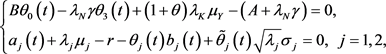$\text{d}G\left(t\right)=G\left(t\right)\left[-r\text{d}t-\underset{j=0}{\overset{2}{\sum }}{\theta }_{j}\left(t\right)\text{d}{W}_{j}\left(t\right)-\underset{j=\text{1}}{\overset{2}{\sum }}{\stackrel{˜}{\theta }}_{j}\left(t\right)\text{d}{B}_{j}\left(t\right)+{\theta }_{3}\left(t\right)\text{d}\stackrel{˜}{N}\left(t\right)\right].$

$Z\left(t\right)=Y\left(t\right)-g\left(t\right),$ (7)

$g\left(t\right)$ 的经济含义表示保险公司即不考虑投资也不考虑再保险。故在数值上，

$g\left(t\right)={\text{e}}^{{\alpha }_{1}rt}\left[C{\int }_{0}^{t}{\text{e}}^{-{\alpha }_{1}rs}\text{d}s-B{\int }_{0}^{t}{\text{e}}^{-{\alpha }_{1}rs}\text{d}{W}_{0}\left(s\right)-{\alpha }_{1}\gamma {\int }_{0}^{t}{\text{e}}^{-{\alpha }_{1}rs}\text{d}\stackrel{˜}{N}\left(s\right)\right]$ (8)

$\begin{array}{c}\text{d}Z\left(t\right)=\left\{r{Z}^{\pi }\left(t\right)-\left({\alpha }_{1}-{\alpha }_{2}\right){\lambda }_{K}{\mu }_{Y}{\pi }_{q}\left(t\right)-\left({\alpha }_{1}-{\alpha }_{2}\right)\theta {\lambda }_{K}{\mu }_{Y}{\pi }_{q}\left(t\right)+\left({\alpha }_{1}-{\alpha }_{2}\right){\pi }_{q}\left(t\right)A\underset{\text{ }}{\overset{\text{ }}{\text{ }}}\\ \text{\hspace{0.17em}}\text{\hspace{0.17em}}+\underset{j=1}{\overset{2}{\sum }}{\alpha }_{j}{\pi }_{j}\left(t\right)\left({\alpha }_{j}\left(t\right)+{\lambda }_{j}{\mu }_{j}-r\right)+\left({\alpha }_{1}-{\alpha }_{2}\right){\lambda }_{N}\gamma {\pi }_{q}\left(t\right)\right\}\text{d}t+\left({\alpha }_{1}-{\alpha }_{2}\right){\pi }_{q}\left(t\right)B\text{d}{W}_{0}\left(t\right)\\ \text{\hspace{0.17em}}\text{\hspace{0.17em}}+\underset{j=1}{\overset{2}{\sum }}{\alpha }_{j}{\pi }_{j}\left(t\right){b}_{j}\text{d}{W}_{j}\left(t\right)-\underset{j=1}{\overset{2}{\sum }}{\alpha }_{j}{\pi }_{j}\left(t\right)\sqrt{{\lambda }_{j}}{\sigma }_{j}\left(t\right)\text{d}{B}_{j}\left(t\right)+\left({\alpha }_{1}-{\alpha }_{2}\right){\pi }_{q}\left(t\right)\gamma \text{d}\stackrel{˜}{N}\left(t\right).\end{array}$

$\begin{array}{c}\text{d}G\left(t\right)Z\left(t\right)=G\left(t\right)\left[\left({\alpha }_{1}-{\alpha }_{2}\right){\pi }_{q}\left(t\right)B-Z\left(t\right){\theta }_{0}\left(t\right)\right]\text{d}{W}_{0}\left(t\right)\\ \text{\hspace{0.17em}}\text{\hspace{0.17em}}+G\left(t\right)\underset{j=1}{\overset{2}{\sum }}\left[{\alpha }_{j}{\pi }_{j}\left(t\right){b}_{j}\left(t\right)-{\theta }_{j}\left(t\right)Z\left(t\right)\right]\text{d}{W}_{j}\left(t\right)\\ \text{\hspace{0.17em}}\text{\hspace{0.17em}}-G\left(t\right)\underset{j=1}{\overset{2}{\sum }}\left[{\alpha }_{j}{\pi }_{j}\left(t\right)\sqrt{{\lambda }_{j}}{\sigma }_{j}\left(t\right)+{\stackrel{˜}{\theta }}_{j}\left(t\right)Z\left(t\right)\right]\text{d}{B}_{j}\left(t\right)\\ \text{\hspace{0.17em}}\text{\hspace{0.17em}}+G\left(t\right)\left[Z\left(t\right){\theta }_{3}\left(t\right)+\left({\alpha }_{1}-{\alpha }_{2}\right){\pi }_{q}\left(t\right)\left(1+{\theta }_{3}\left(t\right)\right)\gamma \right]\text{d}\stackrel{˜}{N}\left(t\right),\end{array}$ (9)

$E\left[G\left(t\right)\delta \right]=y$

$\left\{\begin{array}{l}\underset{\pi \in \Pi }{\mathrm{max}}E\left[U\left(\delta \right)\right]\\ E\left[G\left(T\right)\delta \right]\le y\end{array}$ (10)

${\delta }^{*}=\left\{\begin{array}{l}\xi +\left[y-E\left[G\left(T\right)\xi \right]\right]\frac{G{\left(T\right)}^{\frac{1}{{\gamma }_{1}-1}}}{E\left[G{\left(T\right)}^{\frac{{\gamma }_{1}}{{\gamma }_{1}-1}}\right]},\text{ }\text{ }\text{ }\text{ }\text{ }\text{ }\text{ }\text{ }\text{ }\xi \le Z{\text{e}}^{rT},\\ 0,\text{ }\text{ }\text{ }\text{ }\text{ }\text{ }\text{ }\text{ }\text{ }\text{ }\text{ }\text{ }\text{ }\text{ }\text{ }\text{ }\text{ }\text{ }\text{ }\text{ }\text{ }\text{ }\text{ }\text{ }\text{ }\text{ }\text{ }\text{ }\text{ }\text{ }\text{ }\text{ }\text{ }\text{ }\text{ }\text{ }\text{ }\text{ }\text{ }\text{ }\text{ }\text{ }\text{ }\text{ }\text{ }\text{ }\text{ }\text{ }\text{ }\text{ }\text{ }\text{ }\text{ }\text{ }\text{ }\text{ }\text{ }\text{ }\text{ }\text{ }\text{ }\text{ }\text{ }\text{ }\text{ }\text{ }\text{ }\text{ }\text{ }\text{ }\text{ }\text{ }\text{ }\text{ }\text{ }\text{ }\text{ }\text{ }\text{ }\text{ }\text{ }\text{ }\text{ }\text{ }\text{ }\text{ }\text{ }\text{ }\text{ }\text{ }\text{ }\text{ }\text{ }\text{ }\text{ }\text{ }\text{ }\text{ }\text{ }\text{ }\text{ }\text{ }\text{ }\text{ }\text{ }\text{ }\text{ }\text{ }\text{ }\text{ }\text{ }\text{ }\text{ }\text{ }\text{ }\text{ }\text{ }\text{ }\text{ }\text{ }\text{ }\text{ }\text{ }\text{ }\text{ }\text{ }\text{ }\text{ }\text{ }\text{ }\text{ }\text{ }\text{ }\text{ }\text{ }\text{ }\text{ }\text{ }\text{ }\text{ }\text{ }\text{ }\text{ }\text{ }\text{ }\text{ }\text{ }\xi >Z{\text{e}}^{rT},\end{array}$ (11)

$E\left[G\left(T\right){Z}^{*}\left(T\right)|{F}_{t}\right]=E\left[G\left(T\right)\delta |{F}_{t}\right].$ (12)

$G\left(t\right){Z}^{*}\left(t\right)=\left\{\begin{array}{l}G\left(T\right)\xi +\left[y-E\left[G\left(T\right)\xi \right]\right]\frac{G{\left(T\right)}^{\frac{1}{{\gamma }_{1}-1}}}{E\left[G{\left(T\right)}^{\frac{{\gamma }_{1}}{{\gamma }_{1}-1}}\right]},\text{ }\text{ }\text{ }\text{ }\text{ }\text{ }\text{ }\text{ }\text{ }\text{ }\text{ }\text{ }\text{ }\text{ }\text{ }\text{ }\text{ }\xi \le Z{\text{e}}^{rT}\\ 0,\text{ }\text{ }\text{ }\text{ }\text{ }\text{ }\text{ }\text{ }\text{ }\text{ }\text{ }\text{ }\text{ }\text{ }\text{ }\text{ }\text{ }\text{ }\text{ }\text{ }\text{ }\text{ }\text{ }\text{ }\text{ }\text{ }\text{ }\text{ }\text{ }\text{ }\text{ }\text{ }\text{ }\text{ }\text{ }\text{ }\text{ }\text{ }\text{ }\text{ }\text{ }\text{ }\text{ }\text{ }\text{ }\text{ }\text{ }\text{ }\text{ }\text{ }\text{ }\text{ }\text{ }\text{ }\text{ }\text{ }\text{ }\text{ }\text{ }\text{ }\text{ }\text{ }\text{ }\text{ }\text{ }\text{ }\text{ }\text{ }\text{ }\text{ }\text{ }\text{ }\text{ }\text{ }\text{ }\text{ }\text{ }\text{ }\text{ }\text{ }\text{ }\text{ }\text{ }\text{ }\text{ }\text{ }\text{ }\text{ }\text{ }\text{ }\text{ }\text{ }\text{ }\text{ }\text{ }\text{ }\text{ }\text{ }\text{ }\text{ }\text{ }\text{ }\text{ }\text{ }\text{ }\text{ }\text{ }\text{ }\text{ }\text{ }\text{ }\text{ }\text{ }\text{ }\text{ }\text{ }\text{ }\text{ }\text{ }\text{ }\text{ }\text{ }\text{ }\text{ }\text{ }\text{ }\text{ }\text{ }\text{ }\text{ }\text{ }\text{ }\text{ }\text{ }\text{ }\text{ }\text{ }\text{ }\text{ }\text{ }\text{ }\text{ }\text{ }\text{ }\text{ }\text{ }\text{ }\text{ }\text{ }\text{ }\text{ }\text{ }\text{ }\text{ }\text{ }\text{ }\text{ }\text{ }\text{ }\text{ }\text{ }\text{ }\text{ }\text{ }\text{ }\text{ }\text{ }\text{ }\text{ }\text{ }\text{ }\text{ }\text{ }\text{ }\text{ }\text{ }\text{ }\text{ }\text{ }\xi >Z{\text{e}}^{rT}\end{array}$ (13)

$\begin{array}{c}M\left(t\right)=\mathrm{exp}{\left\{-\underset{j=0}{\overset{2}{\sum }}\frac{1}{2}\left(\frac{{\gamma }_{1}}{{\gamma }_{1}-1}\right)}^{2}{\int }_{0}^{t}{\theta }_{j}^{2}\left(s\right)\text{d}s-\underset{j=0}{\overset{2}{\sum }}\left(\frac{{\gamma }_{1}}{{\gamma }_{1}-1}\right){\int }_{0}^{t}{\theta }_{j}\left(s\right)\text{d}{W}_{j}\left(s\right)\\ \text{\hspace{0.17em}}\text{\hspace{0.17em}}-\underset{j=1}{\overset{2}{\sum }}\frac{1}{2}{\left(\frac{{\gamma }_{1}}{{\gamma }_{1}-1}\right)}^{2}{\int }_{0}^{t}{\stackrel{˜}{\theta }}_{j}^{2}\left(s\right)\text{d}s-\underset{j=1}{\overset{2}{\sum }}\frac{{\gamma }_{1}}{{\gamma }_{1}-1}{\int }_{0}^{t}{\stackrel{˜}{\theta }}_{j}\left(s\right)\text{d}{B}_{j}\left(s\right)\\ \text{\hspace{0.17em}}\text{\hspace{0.17em}}-{\int }_{0}^{t}\left({\left(1+{\theta }_{3}\left(s\right)\right)}^{\frac{{\gamma }_{1}}{{\gamma }_{1}-1}}\right){\lambda }_{N}\text{d}s+\frac{{\gamma }_{1}}{{\gamma }_{1}-1}{\int }_{0}^{t}\mathrm{ln}\left(1+{\theta }_{3}\left(s\right)\right)\text{d}N\left(s\right)\text{ }\text{ }.\end{array}$

$\begin{array}{c}G{\left(t\right)}^{\frac{{\gamma }_{1}}{{\gamma }_{1}-1}}=M\left(t\right)f\left(t\right)\\ =M\left(t\right)\left[\mathrm{exp}\left\{-\frac{{\gamma }_{1}}{{\gamma }_{1}-1}rt+\underset{j=1}{\overset{2}{\sum }}\frac{1}{2}\frac{{\gamma }_{1}}{{\left({\gamma }_{1}-1\right)}^{2}}{\int }_{0}^{t}{\theta }_{j}^{2}\left(t\right)\text{d}s+\underset{j=1}{\overset{2}{\sum }}\frac{1}{2}\frac{{\gamma }_{1}}{{\left({\gamma }_{1}-1\right)}^{2}}\\ \text{\hspace{0.17em}}\text{\hspace{0.17em}}×{\int }_{0}^{t}{\stackrel{˜}{\theta }}_{j}^{2}\left(s\right)\text{d}s+{\int }_{0}^{t}\left({\left(1+{\theta }_{3}\left(s\right)\right)}^{\frac{{\gamma }_{1}}{{\gamma }_{1}-1}}-{\theta }_{3}\left(s\right)-1\right){\lambda }_{N}\text{d}s\right\}\right]\end{array}$

$\frac{E\left[G{\left(T\right)}^{\frac{{\gamma }_{1}}{{\gamma }_{1}-1}}|{F}_{t}\right]}{E\left[G{\left(T\right)}^{\frac{{\gamma }_{1}}{{\gamma }_{1}-1}}\right]}=\frac{E\left[M\left(T\right)f\left(T\right)|{F}_{t}\right]}{f\left(T\right)E\left[M\left(T\right)\right]}=\frac{M\left(t\right)}{M\left(0\right)}=M\left(t\right).$

$\begin{array}{c}\text{d}G\left(t\right){Z}^{*}\left(t\right)=\xi \text{d}G\left(t\right)+\left[y-\xi {\text{e}}^{-rT}\right]\text{d}M\left(t\right)\\ =\xi \left\{G\left(t\right)\left[-r\text{d}t-\underset{j=0}{\overset{2}{\sum }}{\theta }_{j}\left(t\right)\text{d}{W}_{j}\left(t\right)-\underset{j=1}{\overset{2}{\sum }}{\stackrel{˜}{\theta }}_{j}\left(t\right)\text{d}{B}_{j}\left(t\right)+{\theta }_{3}\left(t\right)\text{d}\stackrel{˜}{N}\left(t\right)\right]\right\}\\ \text{\hspace{0.17em}}\text{\hspace{0.17em}}+\left[y-\xi {\text{e}}^{-rT}\right]M\left(t\right)\left[-\underset{j=0}{\overset{2}{\sum }}\frac{{\gamma }_{1}}{{\gamma }_{1}-1}{\theta }_{j}\text{d}{W}_{j}\left(t\right)-\underset{j=1}{\overset{2}{\sum }}\frac{{\gamma }_{1}}{{\gamma }_{1}-1}{\stackrel{˜}{\theta }}_{j}\text{d}{B}_{j}\left(t\right)\right]\\ \text{\hspace{0.17em}}\text{\hspace{0.17em}}-\left({\left(1+{\theta }_{3}\left(t\right)\right)}^{\frac{{\gamma }_{1}}{{\gamma }_{1}-1}}-1\right)\text{d}\stackrel{˜}{N}\left(t\right)\end{array}$

$\begin{array}{l}=-r\xi G\left(t\right)+G\left(t\right)\underset{j=0}{\overset{2}{\sum }}\left[\frac{{\gamma }_{1}}{{\gamma }_{1}-1}{Z}^{{\pi }^{*}}\left(t\right)-\frac{1}{1-{\gamma }_{1}}\xi \right]{\theta }_{j}\left(t\right)\text{d}{W}_{j}\left(t\right)\\ \text{\hspace{0.17em}}\text{\hspace{0.17em}}+G\left(t\right)\underset{j=1}{\overset{2}{\sum }}\left[\frac{{\gamma }_{1}}{{\gamma }_{1}-1}{Z}^{{\pi }^{*}}\left(t\right)-\frac{1}{1-{\gamma }_{1}}\xi \right]{\stackrel{˜}{\theta }}_{j}\left(t\right)\text{d}{B}_{j}\left(t\right)\\ \text{\hspace{0.17em}}\text{\hspace{0.17em}}+G\left(t\right)\left[\left({\left(1+{\theta }_{3}\left(t\right)\right)}^{\frac{{\gamma }_{1}}{{\gamma }_{1}-1}}-1\right)×\left({Z}^{{\pi }^{*}}\left(t\right)-\xi \right)+\xi {\theta }_{3}\left(t\right)\right]\text{d}\stackrel{˜}{N}\left(t\right).\end{array}$ (14)

$\begin{array}{c}\text{d}G\left(t\right){Z}^{{\pi }^{*}}\left(t\right)=G\left(t\right)\left[\left({\alpha }_{1}-{\alpha }_{2}\right){\pi }_{q}\left(t\right)B-{Z}^{{\pi }^{*}}\left(t\right){\theta }_{0}\left(t\right)\right]\text{d}{W}_{0}\left(t\right)\\ \text{\hspace{0.17em}}\text{\hspace{0.17em}}+G\left(t\right)\underset{j=1}{\overset{2}{\sum }}\left[{\alpha }_{j}{\pi }_{j}\left(t\right){b}_{j}\left(t\right)-{\theta }_{j}\left(t\right){Z}^{{\pi }^{*}}\left(t\right)\right]\text{d}{W}_{j}\left(t\right)\\ \text{\hspace{0.17em}}\text{\hspace{0.17em}}-G\left(t\right)\underset{j=1}{\overset{2}{\sum }}\left[{\alpha }_{j}{\pi }_{j}\left(t\right)\sqrt{{\lambda }_{j}}{\sigma }_{j}+{\stackrel{˜}{\theta }}_{j}\left(t\right){Z}^{{\pi }^{*}}\left(t\right)\right]\text{d}{B}_{j}\left(t\right)\\ \text{\hspace{0.17em}}\text{\hspace{0.17em}}+G\left(t\right)\left[{Z}^{{\pi }^{*}}\left(t\right){\theta }_{3}\left(t\right)+\left({\alpha }_{1}-{\alpha }_{2}\right){\pi }_{q}\left(t\right)\left(1+{\theta }_{3}\left(t\right)\right)\gamma \right]\text{d}\stackrel{˜}{N}\left(t\right).\end{array}$ (15)

$\left\{\begin{array}{l}{\pi }_{1}^{*}\left(t\right)=\left[\frac{{a}_{j}\left(t\right)+{\lambda }_{j}{\mu }_{j}-r}{{a}_{j}\left({b}_{j}^{2}\left(t\right)+{\lambda }_{j}{\sigma }_{j}^{2}\right)}+\frac{{\gamma }_{1}}{1-{\gamma }_{1}}\frac{{b}_{j}\left(t\right)-\sqrt{{\lambda }_{j}}{\sigma }_{j}}{{a}_{j}\left({b}_{j}^{2}\left(t\right)+{\lambda }_{j}{\sigma }_{j}^{2}\right)}\right]{Z}^{{\pi }^{*}}\left(t\right)-\frac{1}{1-{\gamma }_{1}}\frac{{b}_{j}\left(t\right)+\sqrt{{\lambda }_{j}}{\sigma }_{j}}{{a}_{j}\left({b}_{j}^{2}\left(t\right)+{\lambda }_{j}{\sigma }_{j}^{2}\right)}\xi ,j=1,\text{2,}\\ {\pi }_{q}^{*}\left(t\right)=\frac{1}{{\alpha }_{2}-{\alpha }_{1}}{\omega }^{*}\left({Z}^{{\pi }^{*}}\left(t\right)-\xi \right).\end{array}$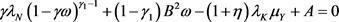(16)

$\begin{array}{l}{Y}^{{\pi }^{*}}\left(t\right)={Z}^{{\pi }^{*}}\left(t\right)+g\left(t\right)\\ =\left\{\begin{array}{l}\xi +g\left(t\right)+\left(y-\xi {\text{e}}^{-rT}\right)\mathrm{exp}\left\{rt-\underset{j=0}{\overset{2}{\sum }}\frac{1}{1-{\gamma }_{1}}{\int }_{0}^{t}{\theta }_{j}\left(s\right)\text{d}{W}_{j}\left(s\right)-\underset{j=0}{\overset{2}{\sum }}\frac{2{\gamma }_{1}-1}{2{\left({\gamma }_{1}-1\right)}^{2}}{\int }_{0}^{t}{\theta }_{j}^{2}\left(s\right)\text{d}s\\ -\underset{j=1}{\overset{2}{\sum }}{\int }_{0}^{t}{\stackrel{˜}{\theta }}_{j}\left(s\right)\text{d}{B}_{j}\left(s\right)-\underset{j=0}{\overset{2}{\sum }}\frac{2{\gamma }_{1}-1}{2{\left({\gamma }_{1}-1\right)}^{2}}{\int }_{0}^{t}{\stackrel{˜}{\theta }}_{j}^{2}\left(s\right)\text{d}s-{\int }_{0}^{t}\left[{\left({\theta }_{3}\left(s\right)+1\right)}^{\frac{{\gamma }_{1}}{{\gamma }_{1}-1}}-{\theta }_{3}\left(s\right)-1\right]{\lambda }_{N}\text{d}s\\ +\frac{1}{{\gamma }_{1}-1}{\int }_{0}^{t}\mathrm{ln}\left(1+{\theta }_{3}\left(s\right)\right)\text{d}N\left(s\right),\text{ }\text{ }\text{ }\text{ }\text{ }\text{ }\text{ }\text{ }\text{ }\text{ }\text{ }\text{ }\text{ }\text{ }\text{ }\text{ }\text{ }\text{ }\text{ }\xi \le y{\text{e}}^{rT},\\ g\left(t\right),\text{ }\text{ }\text{ }\text{ }\text{ }\text{ }\text{ }\text{ }\text{ }\text{ }\text{ }\text{ }\text{ }\text{ }\text{ }\text{ }\text{ }\text{ }\text{ }\text{ }\text{ }\text{ }\text{ }\text{ }\text{ }\text{ }\text{ }\text{ }\text{ }\text{ }\text{ }\text{ }\text{ }\text{ }\text{ }\text{ }\text{ }\text{ }\text{ }\text{ }\text{ }\text{ }\text{ }\text{ }\text{ }\text{ }\text{ }\text{ }\text{ }\text{ }\text{ }\text{ }\text{ }\text{ }\text{ }\text{ }\text{ }\text{ }\text{ }\text{ }\text{ }\text{ }\text{ }\text{ }\text{ }\text{ }\text{ }\text{ }\text{ }\text{ }\text{ }\text{ }\text{ }\text{ }\text{ }\text{ }\text{ }\text{ }\text{ }\text{ }\text{ }\text{ }\text{ }\text{ }\text{ }\text{ }\text{ }\text{ }\text{ }\text{ }\text{ }\text{ }\text{ }\text{ }\text{ }\text{ }\text{ }\text{ }\text{ }\text{ }\text{ }\text{ }\text{ }\text{ }\text{ }\text{ }\text{ }\text{ }\text{ }\text{ }\text{ }\text{ }\text{ }\text{ }\text{ }\text{ }\text{ }\xi >y{\text{e}}^{rT}.\end{array}\end{array}$

$\left\{\begin{array}{l}{\pi }_{1}^{*}\left(t\right)=\left[\frac{{a}_{j}\left(t\right)+{\lambda }_{j}{\mu }_{j}-r}{{a}_{j}\left({b}_{j}^{2}\left(t\right)+{\lambda }_{j}{\sigma }_{j}^{2}\right)}+\frac{{\gamma }_{1}}{1-{\gamma }_{1}}\frac{{b}_{j}\left(t\right)-\sqrt{{\lambda }_{j}}{\sigma }_{j}}{{a}_{j}\left({b}_{j}^{2}\left(t\right)+{\lambda }_{j}{\sigma }_{j}^{2}\right)}\right]{Z}^{{\pi }^{*}}\left(t\right)-\frac{1}{1-{\gamma }_{1}}\frac{{b}_{j}\left(t\right)+\sqrt{{\lambda }_{j}}{\sigma }_{j}}{{a}_{j}\left({b}_{j}^{2}\left(t\right)+{\lambda }_{j}{\sigma }_{j}^{2}\right)}\xi ,\\ {\pi }_{q}^{*}\left(t\right)=\frac{1}{{\alpha }_{2}-{\alpha }_{1}}{\omega }^{*}\left({Z}^{{\pi }^{*}}\left(t\right)-\xi \right).\end{array}$

$\gamma {\lambda }_{N}{\left(1-\gamma \omega \right)}^{{\gamma }_{1}-1}+\left(1-{\gamma }_{1}\right){B}^{2}\omega -\left(1+\eta \right){\lambda }_{K}{\mu }_{Y}+A=0.$

5. 数值分析

$\begin{array}{l}r=0.02,\text{\hspace{0.17em}}\text{\hspace{0.17em}}\eta =0.25,\text{\hspace{0.17em}}\text{\hspace{0.17em}}\theta =0.4,\text{\hspace{0.17em}}\text{\hspace{0.17em}}{\lambda }_{K}=4,\text{\hspace{0.17em}}\text{\hspace{0.17em}}{\lambda }_{N}=1,\text{\hspace{0.17em}}\text{\hspace{0.17em}}{x}_{1}=0.5,\text{\hspace{0.17em}}\text{\hspace{0.17em}}{x}_{2}=0.5,\\ {a}_{1}=0.2,\text{\hspace{0.17em}}\text{\hspace{0.17em}}{b}_{1}=0.3,\text{\hspace{0.17em}}\text{\hspace{0.17em}}{a}_{2}=0.4,\text{\hspace{0.17em}}\text{\hspace{0.17em}}{b}_{2}=0.5,\text{\hspace{0.17em}}\text{\hspace{0.17em}}{\lambda }_{1}=2,\text{\hspace{0.17em}}\text{\hspace{0.17em}}{\mu }_{1}=0.2,\text{\hspace{0.17em}}\text{\hspace{0.17em}}{\lambda }_{2}=2,\text{ }\\ {\mu }_{2}=0.2,\text{\hspace{0.17em}}\text{\hspace{0.17em}}{\sigma }_{1}=0.1,\text{\hspace{0.17em}}\text{\hspace{0.17em}}{\sigma }_{2}=0.2,\text{\hspace{0.17em}}\text{\hspace{0.17em}}\gamma =0.001,\text{\hspace{0.17em}}\text{\hspace{0.17em}}{\mu }_{Y}=\frac{1}{40},\text{\hspace{0.17em}}\text{\hspace{0.17em}}{\sigma }_{Y}=\frac{1}{{40}^{2}}.\end{array}$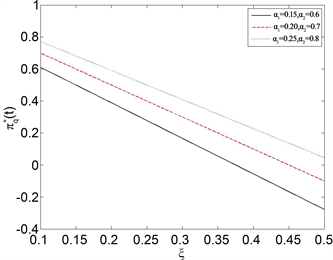Figure 1. The effect ofon the optimal reinsurance strategy ${\pi }_{q}^{*}\left(t\right)$ when ${\alpha }_{1}<{\alpha }_{2}$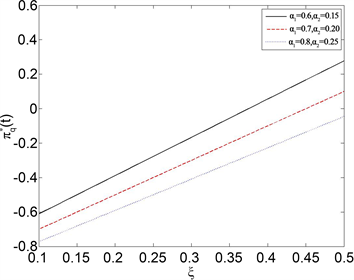Figure 2. The effect of $\xi$ on the optimal reinsurance strategy ${\pi }_{q}^{*}\left(t\right)$ when ${\alpha }_{1}>{\alpha }_{2}$Figure 3. The effect of ${a}_{1}$ on the optimal reinsurance strategy ${\pi }_{\text{1}}^{*}\left(t\right)$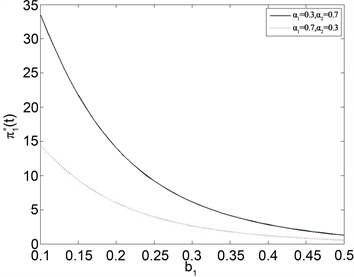Figure 4. The effect of ${b}_{1}$ on the optimal reinsurance strategy ${\pi }_{\text{1}}^{*}\left(t\right)$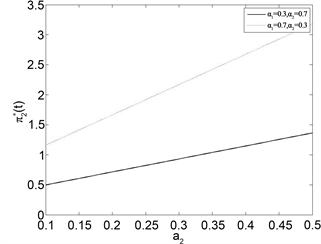Figure 5. The effect of ${a}_{2}$ on the optimal reinsurance strategy ${\pi }_{\text{2}}^{*}\left(t\right)$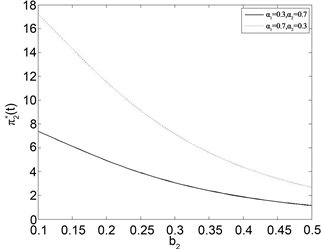Figure 6. The effect of ${b}_{2}$ on the optimal reinsurance strategy ${\pi }_{\text{2}}^{*}\left(t\right)$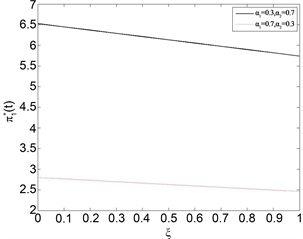Figure 7. The effect of $\xi$ on the optimal reinsurance strategy ${\pi }_{\text{1}}^{*}\left(t\right)$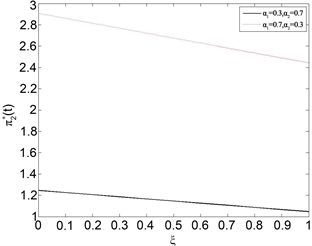Figure 8. The effect of $\xi$ on the optimal reinsurance strategy ${\pi }_{2}^{*}\left(t\right)$

6. 总结

  Zhang, Q. and Chen, P. (2019) Robust Optimal Proportional Reinsurance and Investment Strategy for an Insurer with Defaultable Risks and Jumps. Journal of Computational and Applied Mathematics, 356, 46-66. https://doi.org/10.1016/j.cam.2019.01.034  Yang, H.L. and Zhang, L.H. (2005) Optimal Investment for Insurer with Jump-Diffusion Risk Process. Insurance Mathematics and Economics, 37, 615-634. https://doi.org/10.1016/j.insmatheco.2005.06.009  Browne, S. (1995) Optimal Investment Policies for a Firm with Random Risk Process: Exponential Utility and Minimizing the Probability of Ruin. Mathematics of Operations Research, 20, 937-958. https://doi.org/10.1287/moor.20.4.937  Bai, L.H. and Guo, J.Y. (2008) Optimal Proportional Reinsurance and Investment with Multiple Risky Assets and No-Shorting Constraint. Insurance Mathematics and Economics, 42, 968-975. https://doi.org/10.1016/j.insmatheco.2007.11.002  Zhang, X., Meng, H. and Zenf, Y. (2016) Optimal Investment and Reinsurance Strategies for Insurers with Generalized Mean-Variance Premium Principle and No-Short Selling. Insurance Mathematics and Economics, 67, 125-132. https://doi.org/10.1016/j.insmatheco.2016.01.001  Shen, Y. and Zeng, Y. (2015) Optimal Invest-ment-Reinsurance Strategy for Mean-Variance Insurers with Square-Root Factor Process. Insurance Mathematics and Economics, 62, 118-137. https://doi.org/10.1016/j.insmatheco.2015.03.009  Wu, H.L. and Zeng, Y. (2015) Equilibrium Investment Strategy for Deined-Contribution Pension Schemes with Generalized Mean-Variance Criterion and Mortality Risk. Insurance Mathematics and Economics, 64, 396-408. https://doi.org/10.1016/j.insmatheco.2015.07.007  Zhang, C.B. and Liang, Z.B. (2016) Portfolio Optimization for Jump-Diffusion Risky Assets with Common Shock Dependence and State Dependent Risk Aversion. Optimal Control Applications and Methods, 38, 229-246. https://doi.org/10.1002/oca.2252  Tversky, A. and Kahnean, D. (1992) Advances in Prospect Theory: Cumulative Representation of Uncertainty. Journal of Risk and Uncertainty, 5, 297-323. https://doi.org/10.1007/BF00122574  Berkelaar, A.B., Kouwenberg, R. and Post, T. (2004) Optimal Portfolio Choice under Loss Aversion. Review of Economics and Statistics, 86, 973-987. https://doi.org/10.1162/0034653043125167  Dong, Y.H. and Zheng, H. (2019) Optimal Investment of DC Pension Plan under Short-Selling Constraints and Portfolio Insurance. Insurance Mathematics and Economics, 85, 47-59. https://doi.org/10.1016/j.insmatheco.2018.12.005  郭文旌. 基于损失规避行为的最优保险投资与再保险策略选择[J]. 系统科学与数学, 2018, 38(9): 1005-1017.  Oksendal, B. and Sulem, A. (2005) Applied Stochastic Control of Jump Diffusions. Springer, Berlin.  Guo, W.J. (2014) Optimal Portfolio Choice for an Insurer with Loss Aversion. Insurance Mathematics and Economics, 58, 217-222. https://doi.org/10.1016/j.insmatheco.2014.07.004